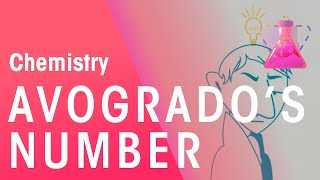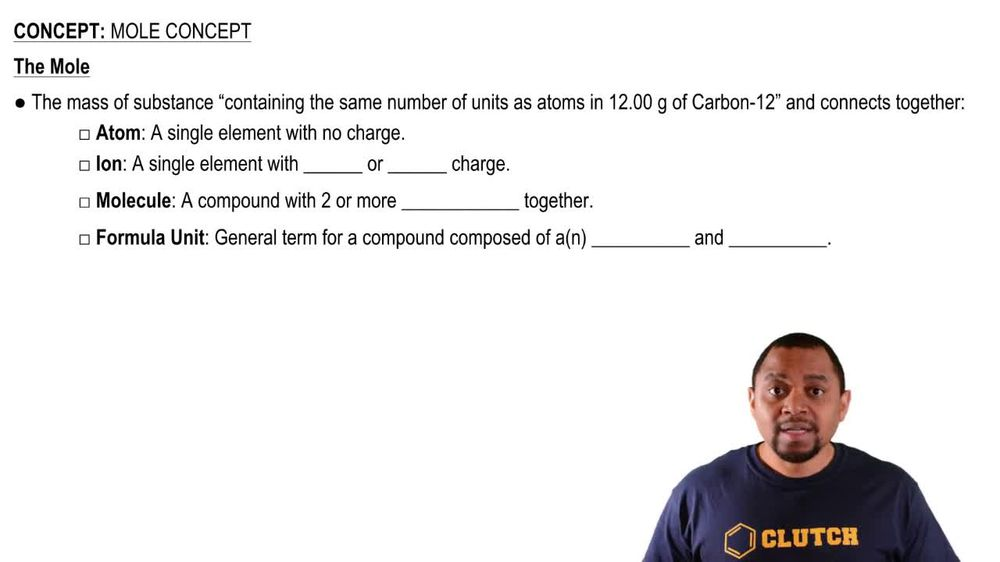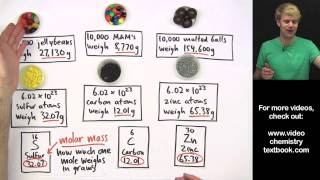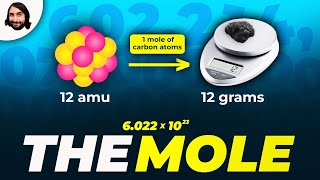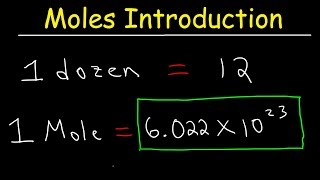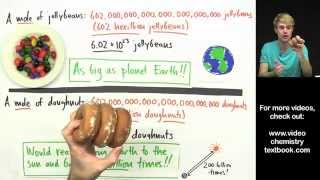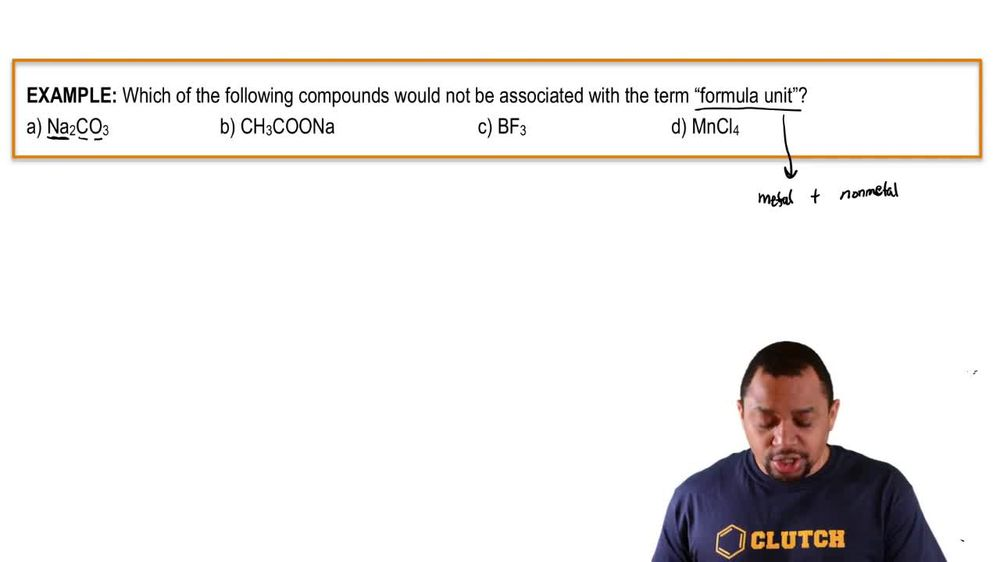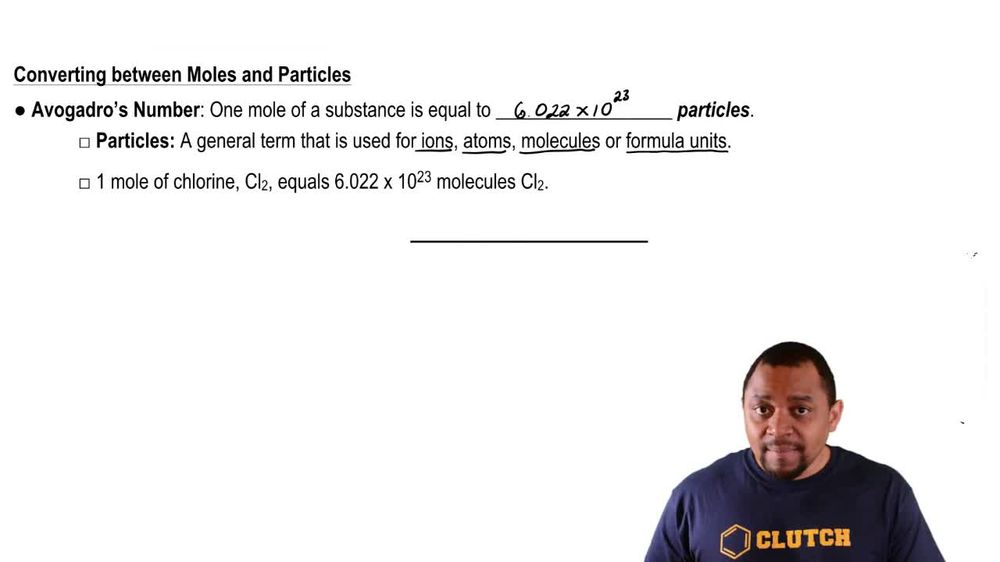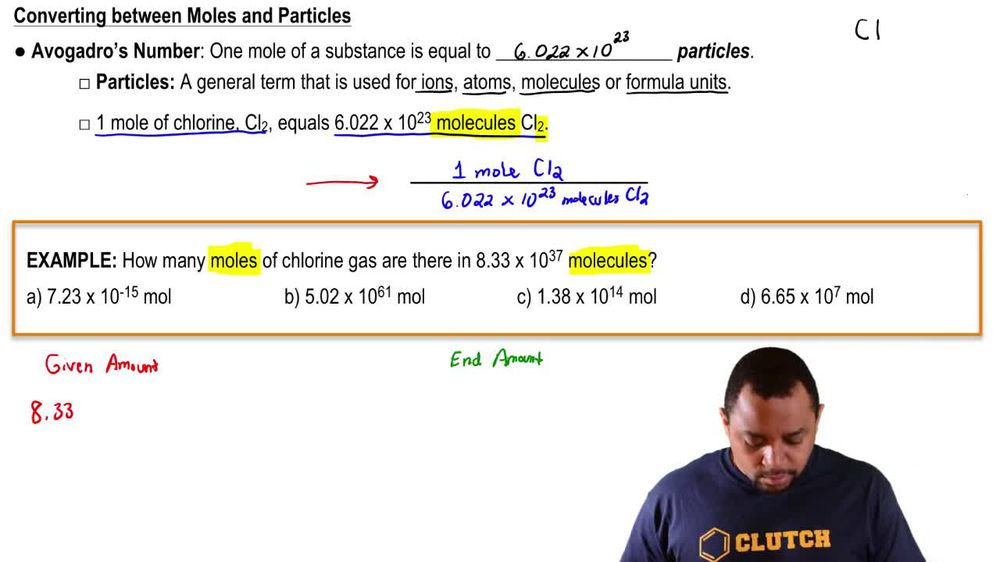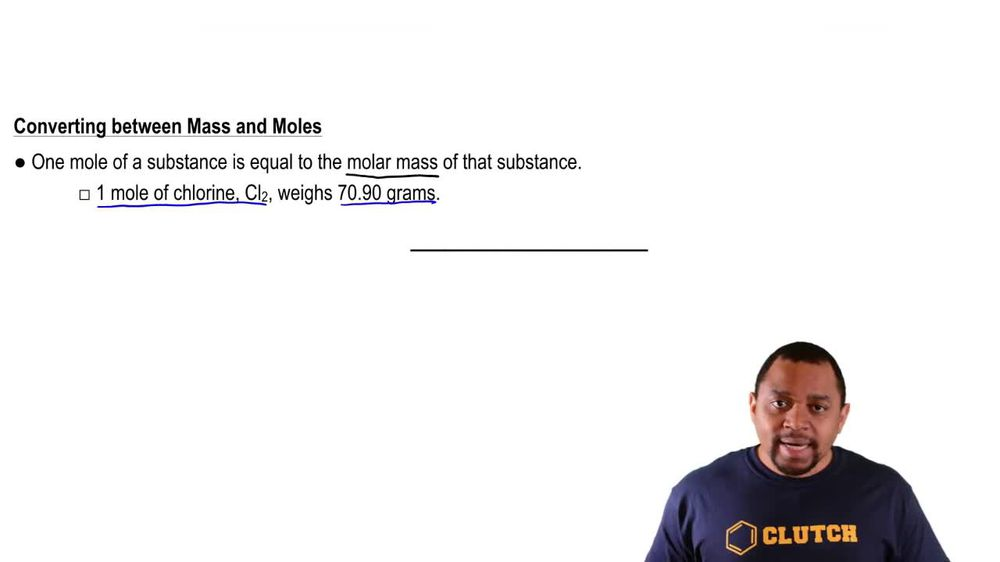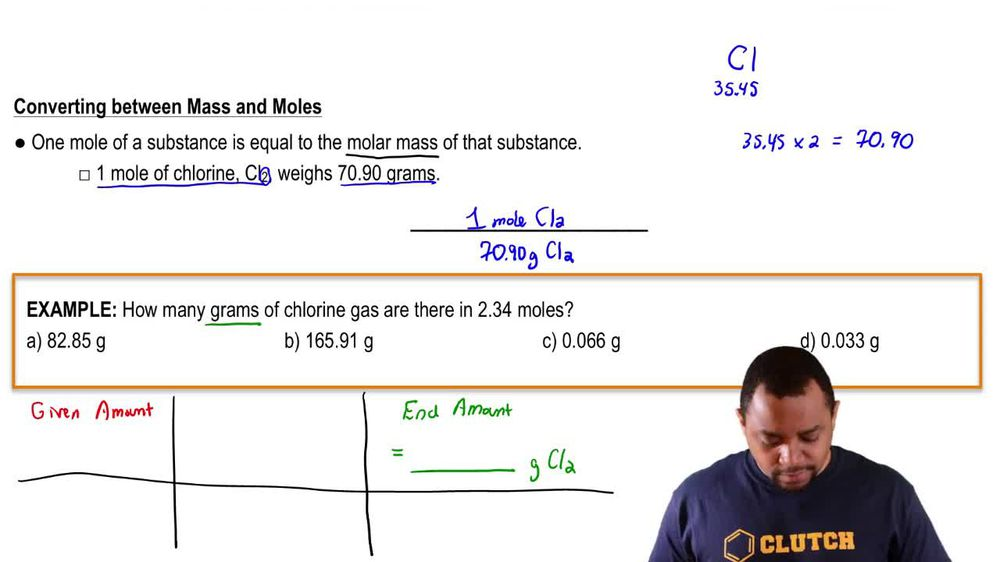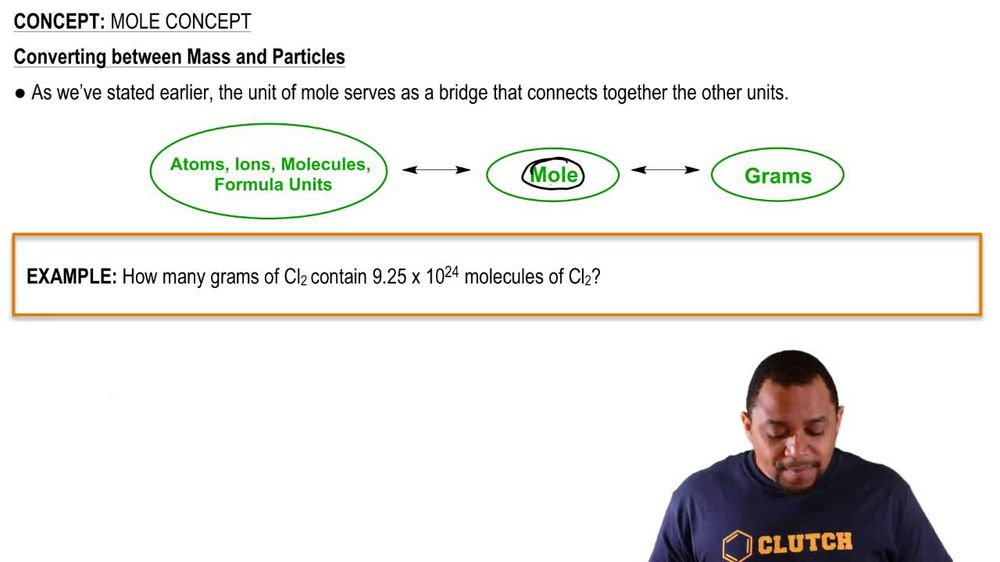Start typing, then use the up and down arrows to select an option from the list.
1. 2. Atoms & Elements2. Mole Concept
Problem

# How many fluorine atoms are present in 5.85 g of C2F4?

Relevant Solution2m
Play a video:
Hey everyone welcome back. So let's get started with this video. So here they want us to calculate the number of carbon atoms in 26. g of butane And we're told the mother mass of butane is 58. g per mole. Okay, so here we're told that there's 26.3 g of butane. Therefore that's going to be what we're going to start with. So it's going to be grams. Then from grams are going to want to go to moles And how are we going to get 2Molds? That is by using the molar mass of beauty in which we're actually told it's 58 .1 g per mole. Okay. And then for most we're going to want to find the moles of carbon in the formula of butane. Once again, we're going to look here and that's how we're going to find the moles of carbon. And then finally we're going to go to the number of carbon atoms. So Adams and we're going to go to their using avocados number Which is 6.02, 2 times 10 to the 23. Okay, so let's go ahead and start our store kilometer. So then we're going to start with the Graham's given, which is 26 0.3 grams of butane C four H 10. Okay, so here we're going to use the molar mass, which we're told is 58 .1g of beauty for one more C four H 10. Okay, so then for moles, we're going to find the moles of carbon. So then one more beauty. See for age 10 contains four moles of carbon. So four months. See, okay, finally we're going to go to atoms by using over gorgeous number. So then one more carbon is 6.0-2 Times 10 to the Adams of carbon. So then grams cancel out moles, cancel out moles of carbon cancel and we get Adams of carbon. We do the math and it's one 09 times 10 To the 24 atoms of carbon. Okay, thank you for watching. I hope this helped. And I'll see you in the next video.